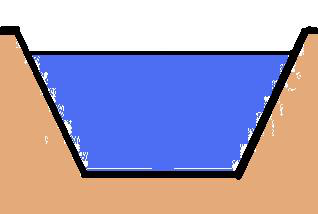* Log In to use the Calculate function * Become a Member!

###### Channel Design:Velocity (V)
• Calculate the velocity (V) of uniform flow in an open channel.
Flow (Q)
• Calculate the flow volume (Q) of uniform flow in an open channel.
Flow Depth (d)
• Calculate the depth (d) of uniform flow in an open channel.
Critical Depth (dc)
• Calculate the critical depth (dc) for a flow volume (Q) for an open channel cross section shape.
Channel Bend Depth
• Calculate the difference in water surface elevations (channel depth) between the inner and outer banks of a bend in an open channel.
Froude No. - Rectangular
• Calculate the Froude number for an open channel with a rectangular cross section.
Froude No. - Other
• Calculate the Froude number for any open channel.
Rectangular Weir
• Calculate the discharge or flow volume (Q) through a rectangular weir.
Rectangular Weir (Contracted)
• Calculate the discharge or flow volume (Q) through a contracted rectangular weir.
Triangular Weir (V-notch)
• Calculate the discharge or flow volume (Q) through a triangular (V-notch) weir.
Trapezoidal (Cipoletti) Weir
• Calculate the discharge or flow volume (Q) through a trapezoidal (Cipoletti) weir.
Parshall Flume
• Calculate the discharge or flow volume (Q) through a Parshall flume.
Design Problem 1
• Open Channels Design Problem 1 - Determine the depth of flow, critical depth and if flow is subcritical or supercritical for a grass lined trapezoidal channel.

 Start Using The Calculations >>> Become A Member Now!